# From Attention to Self Attention to Transformers

The Transformer model uses a “Scaled Dot Product” attention mechanism. The transformer model also uses what is called as “Multi-Head Attention” — instead of calculating just one attention score for a given input, multiple attention scores are calculated — using different sets of weights. This allows the model to attend to different “representation sub-spaces” at different positions, akin to using different filters to create different features maps in a single layer in a CNN.

This is really a continuation of an earlier post on “Introduction to Attention”, where we saw some of the key challenges that were addressed by the attention architecture introduced there (and referred in Fig 1 below).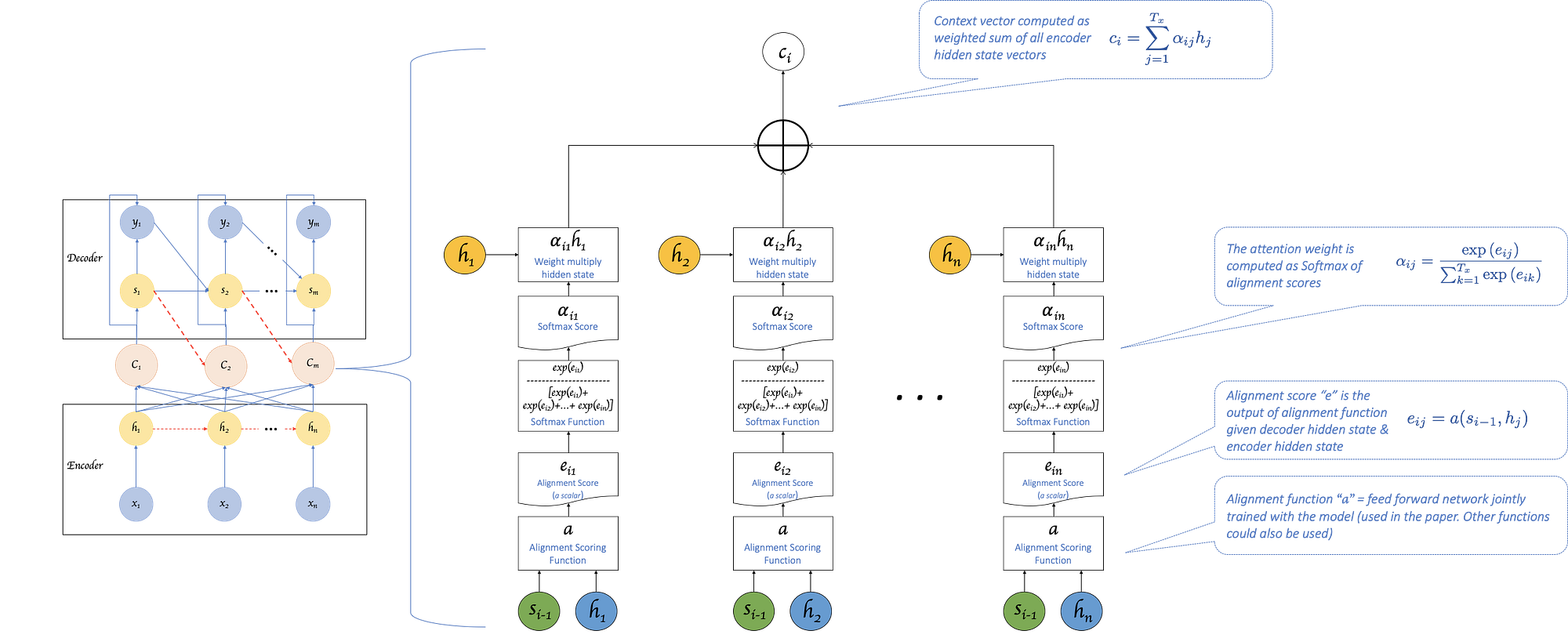Fig 1: From “Introduction to Attention”, based on paper by Bahdanau et al.

The challenge of one single context vector, the final hidden state in the encoder RNN, holding the meaning of the entire sentence/input sequence was addressed by replacing that with an attention based context vector generated for every decoder step as seen in Figure 1.

But it introduced the challenge of increased computational complexity of computing a separate context vector for every step of decoder. And this was over and above the already existing parallelization related challenge. i.e. The sequential nature of processing that is required in RNNs — give that hidden state h1 is required to compute the next hidden state h2, these operations cannot be done in parallel. Both of these challenges are represented by the dashed red lines in the left half of Figure 1 (and also called out in Figure 3).

Talking about sequential processing, you might also be wondering, given that we are replacing the one final hidden state with a context vector generated for every output step — do we need the “h” states at all? After all, attention alignment is supposed to define which part of the input the given output step should focus on, and “h” is only an indirect representation of “x”. It represents the context of all input steps until “x” and not just “x” alone. Wouldn’t using “x” directly make more sense?

Turns out it does.

End to End Memory networks introduced in this paper by Sukhbaatar et alproposes. Pasted in Figure 2 is just a single layer version of the proposed model. The proposed model has “input memory” or “key” vectors representing all inputs, a “query” vector to which the model needs to respond to (like the last decoder hidden state) and “value” or “output memory” vectors — again a representation of the inputs. The inner product between “query” and “keys” give the “match” (akin to attention) probability. The sum of “value” vectors weighted by the probability gives the final response. While producing good results, this eliminated sequential processing of the inputs and replaced it with a “memory query” paradigm.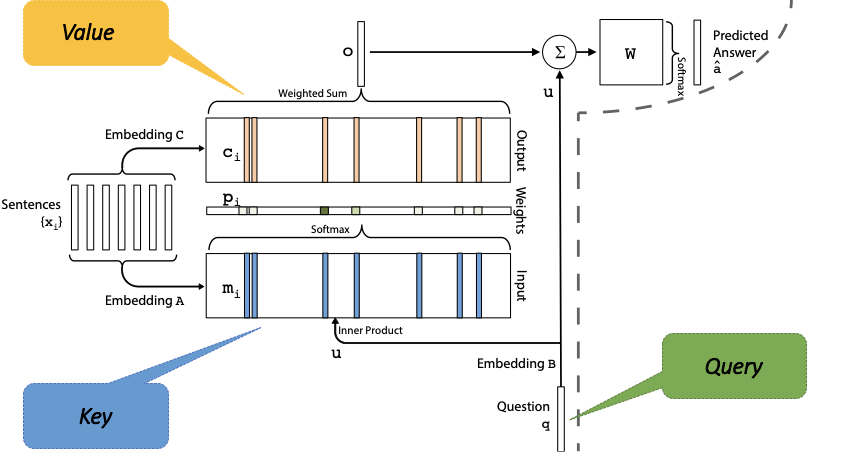Fig 2: End to End Memory Networks by Sukhbaatar et al

Compare this with the base attention model we have seen earlier and the “similarities” will start to emerge. While there are differences between the two — “End to End Memory Networks” proposed a memory across sentences and multiple “hops” to generate an output, we can borrow the concepts of “Key”, “Query” and “Value” to get a generalized view of our base model. Figure 3 calls out these concepts as it applies to the base model.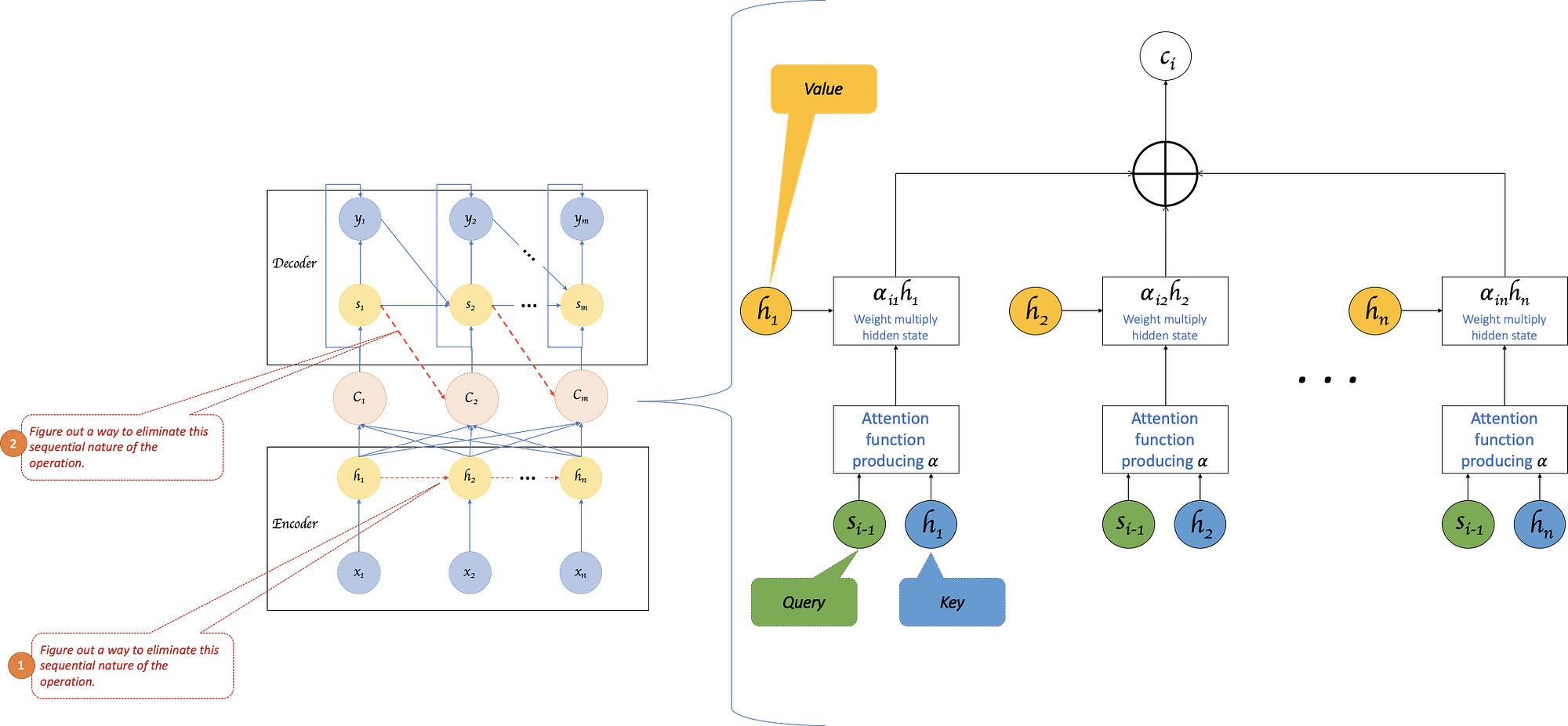Fig 3: Challenges in the attention model from “Introduction to Attention” based on paper by Bahdanau et al to Transformers

Figure 3 also highlights the two challenges we would love to resolve. For challenge #1, we could perhaps just replace the hidden state (h) acting as keys with the inputs (x) directly. But this wouldn’t be a rich representation – if we directly use word embeddings. The end-to-end memory network used different embedding matrices for input and output memory representations, which is better but they are still independent representations of the word. Compare this with the hidden state (h) which represents not just the word, but the word in context of the given sentence.

Is there a way to eliminate the sequential nature of generating hidden states, but still produce a richer, context representing vector?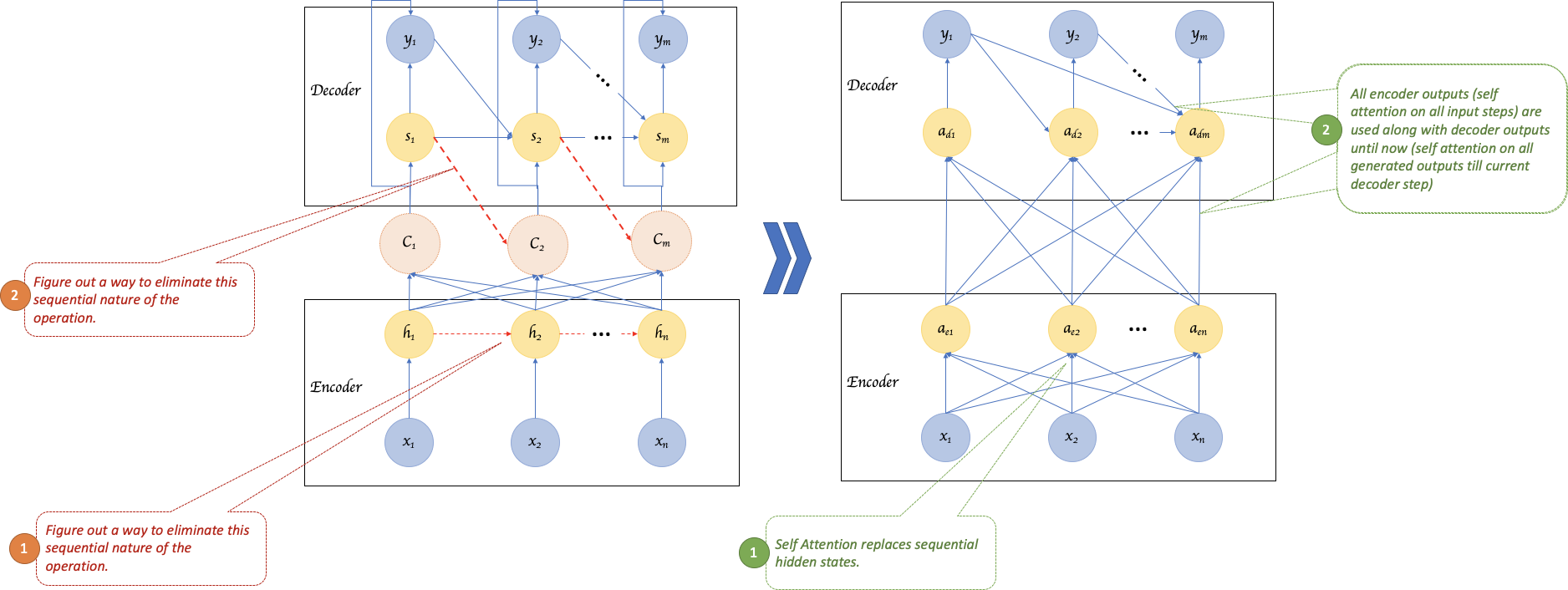Fig 4: Sequence to Sequence with self attention — Moving from being “RNN based” to “attention only”

Figure 4 illustrates a possible way to do this. What if, instead of using attention to connect encoder and decoder, we use attention within encoder and decoder respectively? Attention, after all, is a rich representation — as it considers all keys and values. So instead of deriving hidden states using a RNN, we can use an attention based replacement where inputs (x) are used as “Keys” and “Values”. (i.e. “h”s are replaced by “x”s as illustrated in Figure 5 below) ?

On the encoder side, we can use self attention to generate a richer representation of a given input step xi, with respect to all other items in the input x1, x2…xn. This can be done for all input steps in parallel, unlike hidden state generation in a RNN based encoder. We are basically moving the criss-crossed lines on the left half of Figure 4 downwards as seen in the right half, thereby eliminating the dashed red lines between the representative vectors.

On the decoder side, we can do something similar. We replace a RNN based decoder to an attention based decoder. i.e. there are no hidden states anymore and no computation of a separate context vector for every decoder step. Instead, we do self attention on all outputs generated so far and along with it consume the entirety of encoder output. In other words, we are applying attention to whatever we know so far. (Side note — this is strictly not how it happens in Transformers, where attention over generated outputs and encoder output are done in two separate layers one after the other).

The “Transformer” model, introduced in the paper “Attention Is All You Need” by Vaswani et al. and seen in Figure 6 below, does what we discussed above.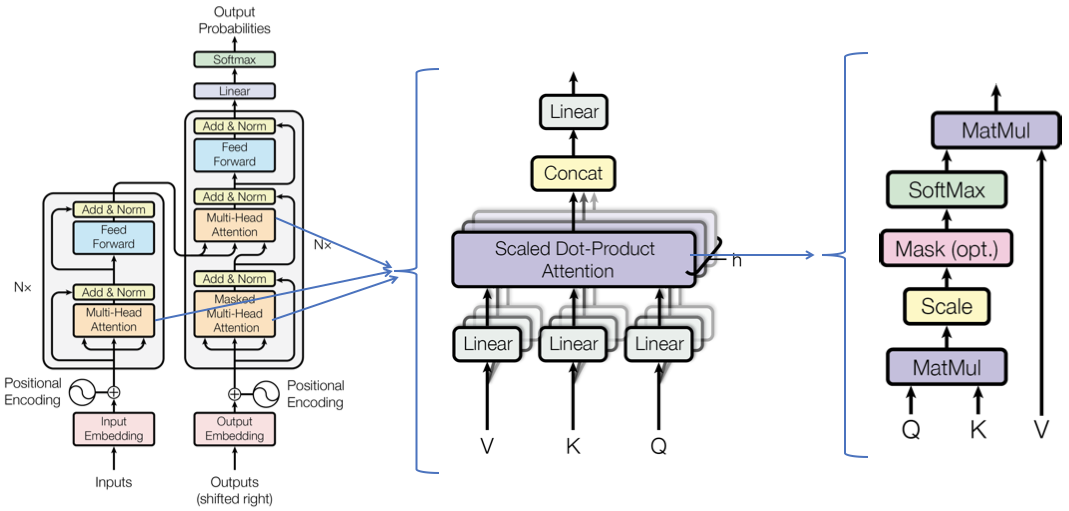Fig 6: From “Attention Is All You Need” by Vaswani et al.

The Transformer model uses a “Scaled Dot Product” attention mechanism. It is illustrated in right side of Figure 6 and also in Figure 7. Compare Figure 7 and Figure 1 to get a sense of differences in “how” attention is computed between the two models. (Note: the “where” also differs, we’ll get to that next). The transformer model also uses what is called as “Multi-Head Attention” — instead of calculating just one “ai” (attention) for a given “xi”, multiple attention scores “ai”s are calculated — using different sets of Ws. This allows the model to attend to different “representation sub-spaces” at different positions, akin to using different filters to create different features maps in a single layer in a CNN.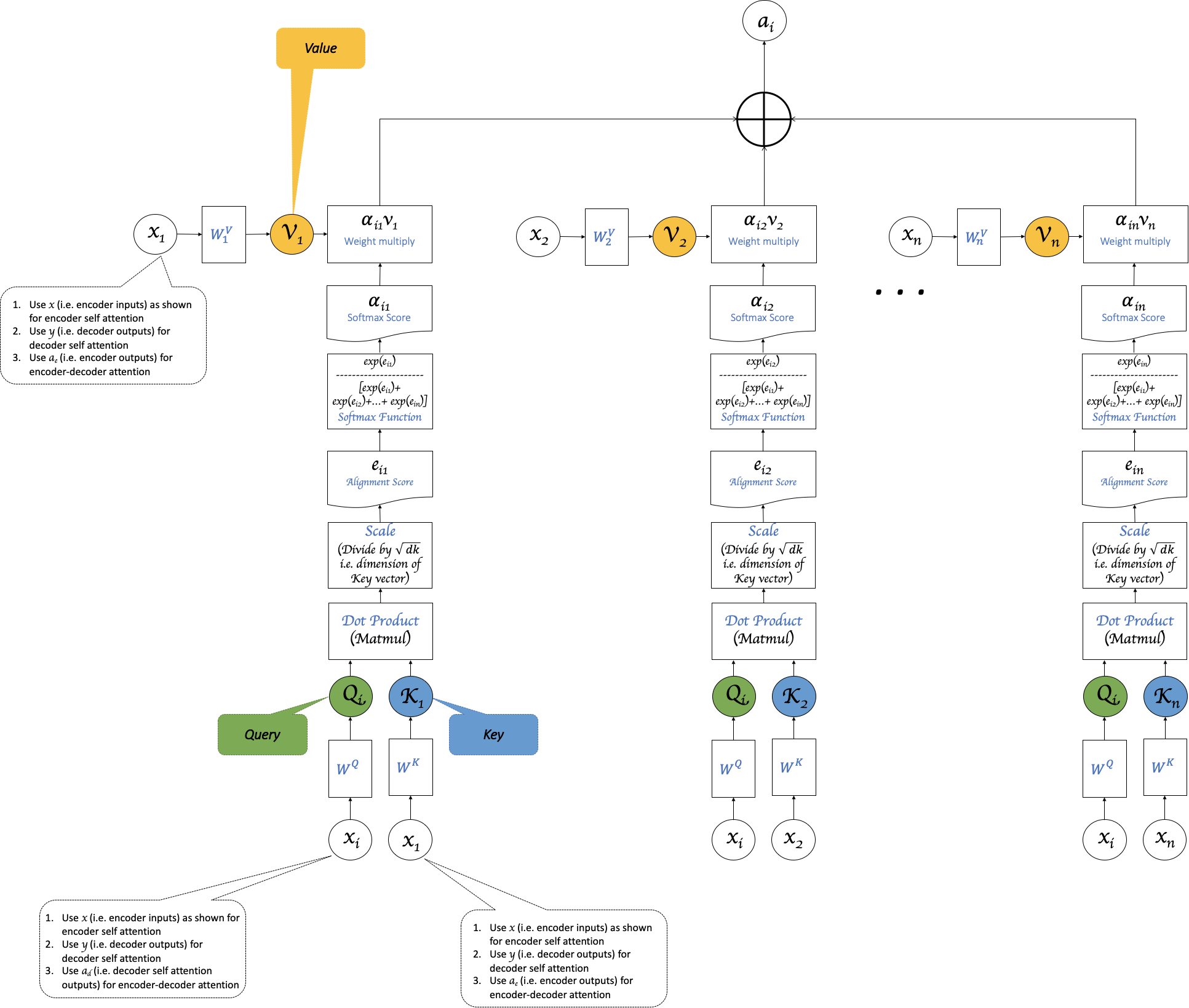Fig 7: Scaled Dot Product used in “Attention Is All You Need” by Vaswani et al.

Encoder

The encoder in the proposed Transformer model has multiple “encoder self attention” layers. Each layer is constructed as follows:

1. The input will be the word embeddings for the first layer. For subsequent layers, it will be the output of previous layer.
2. Inside each layer, first the multi-head self attention is computed using the inputs for the layer as keys, queries and values.
3. The output of #2 is sent to a feed-forward network layer. Here every position (i.e. every word representation) is fed through the same feed-forward that contains two linear transformations followed by a ReLU (input vector ->linear transformed hidden1->linear transformed hidden2 ->ReLU output).

Decoder

The decoder will also have multiple layers. Each layer is constructed as follows:

1. The input will be the word embeddings generated so far for the first layer. For subsequent layers, it will be the output of previous layer.
2. Inside each layer, first the multi-head self attention is computed using the inputs for the layer as keys, queries and values (i.e. generated decoder outputs so far, padded for rest of positions).
3. The output of #2 is sent to a “multi-head-encoder-decoder-attention” layer. Here yet another attention is computed using #2 outputs as queries and encoder outputs as keys and values.
4. The output of #3 is sent to a position wise feed-forward network layer like in encoder.

While getting rid of the sequential nature was helpful in many ways, it took of one key advantage — of knowing the order of words in the input sequence. Without it, the same word occurring in different positions within the same sentence might end up with the same output representation (since it will have the same key, value etc). So the model uses “Positional Encodings” — basically a vector that represents position which is added to the input embeddings at the bottom of the encoder and decoder stack. There’s another paper by Shaw et al here that proposes a relational position based alternative that achieves better result than absolute positional encoding suggested in the original Transformer model paper — I recommend looking into that if you can spend time on the positional embeddings.

Hope this was helpful in some way.